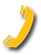top of pagePrivate Golf Lesson

• 1 (one) private 50 minute golf lesson

\$65.00

Series of 3 Private Golf Lessons

•  3(three) private 50 minute golf lesson

\$165.00

Series of 5 Private Golf Lessons

• 5 (five) private 50 minute golf lessons

\$250.00

Semi-Private Golf Lesson- 2 Students

• 1 (one) hour semi-private golf lesson

• 2( two) students

\$40.00/Student

Semi-Private Golf Lesson - 3 Students

• 1(one) hour semi-private golf lesson

• 3 (three) students

\$30.00/Student

Semi-Private Golf Lesson - 4 Students

• 1 ( one) hour semi-private golf lesson

• 4( four) students

\$25.00/Student

2014 Seasonal Program

• 8 half ( 1/2) hour private golf lessons

• Practice privileges after golf lessons

• V1 swing analysis

• TOMI putting analysis

• Weekly practice plans

• 20% discount on schools

• Golf Fitness Session with certified TPI Trainer

\$340.00